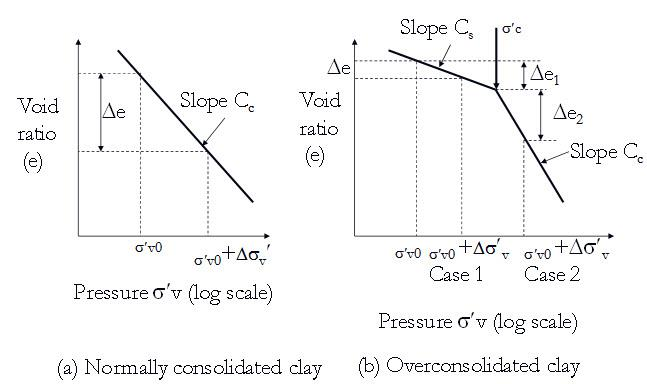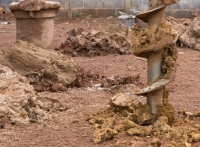Courses

# Settlement Calculation - Consolidation Test - Consolidation of Soil, Soil Mechanicses Agricultural Engineering Notes | EduRev

## Agricultural Engineering: Settlement Calculation - Consolidation Test - Consolidation of Soil, Soil Mechanicses Agricultural Engineering Notes | EduRev

The document Settlement Calculation - Consolidation Test - Consolidation of Soil, Soil Mechanicses Agricultural Engineering Notes | EduRev is a part of the Agricultural Engineering Course Soil Mechanics Notes- Agricultural Engineering.
All you need of Agricultural Engineering at this link: Agricultural Engineering

Settlement Calculation

Figure 23.5 shows the e – log σv' plots for normally consolidated and overconsolidated clay for settlement calculation. For normally consolidated clay the settlement (S) can be determined as:

$S={{{C_c}} \over {1 + {e_0}}}H{\log _{10}}\left( {{{{{\sigma '}_{v0}} + \Delta {{\sigma '}_v}} \over {{{\sigma '}_{v0}}}}} \right)$                    (23.4)

where H is total thickness of the soil layer.

For over-consolidated clay:  Case 1: σ'v0+Δσ'v < σ'c

$S={{{C_s}} \over {1 + {e_0}}}H{\log _{10}}\left( {{{{{\sigma '}_{v0}} + \Delta {{\sigma '}_v}} \over {{{\sigma '}_{v0}}}}} \right)$                       (23.5)

Case 2: σ'v0 < σ'c < σ'v0+Δσ'v

$S={{{C_s}} \over {1 + {e_0}}}H{\log _{10}}\left( {{{{{\sigma '}_c}} \over {{{\sigma '}_{v0}}}}} \right)$   +  ${{{C_c}} \over {1 + {e_0}}}H{\log _{10}}\left( {{{{{\sigma '}_{v0}} + \Delta {{\sigma '}_v}} \over {{{\sigma '}_c}}}} \right)$                           (23.6)

where Cs is the swelling index.Fig. 23.5. Settlement calculation (a) Normally consolidated clay

(b)  Overconsolidated clay .

The document Settlement Calculation - Consolidation Test - Consolidation of Soil, Soil Mechanicses Agricultural Engineering Notes | EduRev is a part of the Agricultural Engineering Course Soil Mechanics Notes- Agricultural Engineering.
All you need of Agricultural Engineering at this link: Agricultural EngineeringUse Code STAYHOME200 and get INR 200 additional OFF Use Coupon Code
All Tests, Videos & Notes of Agricultural Engineering: Agricultural Engineering## Soil Mechanics Notes- Agricultural Engineering

64 docs

Track your progress, build streaks, highlight & save important lessons and more!

,

,

,

,

,

,

,

,

,

,

,

,

,

,

,

,

,

,

,

,

,

,

,

,

;